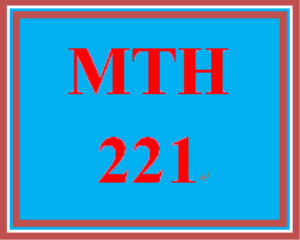# MTH 221 Wk 5 - Final Exam

PLDZ-13191 Free In Stock
\$ 0.00 USD
Description

***************************************************************

~~~~~~~~~~~~~~~~~~~~~~~~~~~~~~~~~~~~~~~~~~~~~~~~~

https://uopcourse.com/

***************************************************************

MTH 221 Wk 5 - Final Exam

Complete the final exam. You have one attempt at the exam. So, be sure to review all previous course materials before attempting the exam.

Question 1

Select the correct value for 74mod11.

3

8

-3

-8

Question 2

What is the value of left parenthesis 260 times 5321 plus 42 times 28 right parenthesis mod 13 ?

6

7

9

12

Question 3

x equals 3 to the power of 4 times 5 cubed times 7 times 11 to the power of 4 y equals 3 squared times 5 to the power of 4 times 7 times 13 to the power of 4

What is the prime factorization for gcd(x, y)?

3 squared times 5 cubed

3 squared times 5 cubed times 7

3 squared times 5 cubed times 7 squared

3 squared times 5 cubed times 7 times 11 to the power of 4 times 13 to the power of 4

Question 4

Let straight pi left parenthesis x right parenthesis be the number of prime numbers in the range from 2 to x. Select the pair of inequalities that are both true.

straight pi left parenthesis 1000 right parenthesis less or equal than straight pi left parenthesis 10000 right parenthesis fraction numerator straight pi left parenthesis 1000 right parenthesis over denominator 1000 end fraction less or equal than fraction numerator straight pi left parenthesis 10000 right parenthesis over denominator 10000 end fraction

straight pi left parenthesis 1000 right parenthesis less or equal than straight pi left parenthesis 10000 right parenthesis fraction numerator straight pi left parenthesis 1000 right parenthesis over denominator 1000 end fraction greater or equal than fraction numerator straight pi 10000 right parenthesis over denominator 10000 end fraction

straight pi left parenthesis 1000 right parenthesis greater or equal than straight pi left parenthesis 10000 right parenthesis fraction numerator straight pi left parenthesis 1000 right parenthesis over denominator 1000 end fraction less or equal than fraction numerator straight pi left parenthesis 10000 right parenthesis over denominator 10000 end fraction

straight pi left parenthesis 1000 right parenthesis greater or equal than straight pi left parenthesis 10000 right parenthesis fraction numerator straight pi left parenthesis 1000 right parenthesis over denominator 1000 end fraction greater or equal than fraction numerator straight pi left parenthesis 10000 right parenthesis over denominator 10000 end fraction

Question 5

Use the equation below to determine the multiplicative inverse of 23 mod 96.

1 equals 6 times 96 minus 25 times 23

6

25

-25

71

Question 6

Select the decimal representation for (A07)16 .

261

263

2560

2567

Question 7

Select the correct value for 740mod2399 . The following equalities may be useful:

72mod2399=49

74mod2399=2

78mod2399=4

716mod2399=16

732mod2399=256

764mod2399=512

260

514

1024

2048

Question 8

Alice encrypts a message m to send to Bob by computing:

(m + 51) mod 83.

If the cyphertext that Alice sends is 11, then what was the original message?

Recent Reviews Write a Review
0 0 0 0 reviews